### Home > PC > Chapter 4 > Lesson 4.2.2 > Problem4-89

4-89.
1.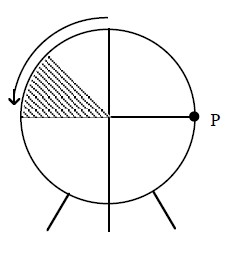On the midway of MathAmerica Carnival land is a wheel of fortune. A transparent wheel with a radius of one meter is in front of a shaded winning region that is stationary behind the wheel. Spin the wheel and if point P lands in the region, you win. Homework Help ✎

1. If the point moves 5 radians around the circle, how many degrees has it moved?

2. Show the new position of the point after the rotation in part (a).

3. The point has traveled a total of 1200 degrees. How far has it traveled?

4. If the point rests in the shaded region when the wheel stops, the player wins. Will the player win?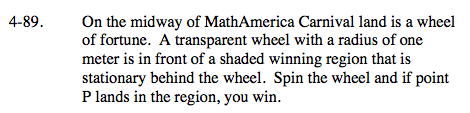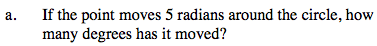$\frac{\text{degrees}}{180\degree}=\frac{\text{radians}}{\pi}$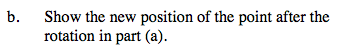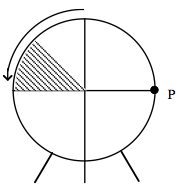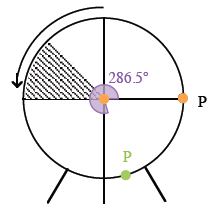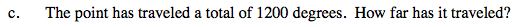$\frac{1200}{360}=\text{ number of times around + the remainder.}$

$\text{Circumference }=(2\pi)\text{radius}$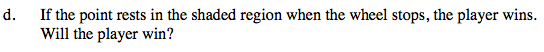That is short of the shaded region.